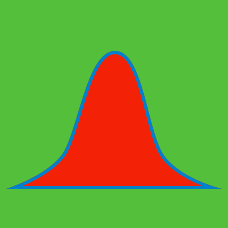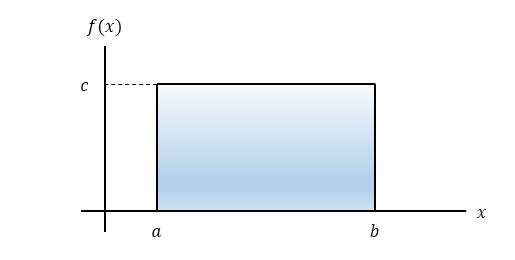Probability

# Continuous Probability Distributions - Uniform Distribution

$X$ is a random variable that follows a continuous uniform distribution with probability density function $f(x) = \left\{\begin{matrix} \frac{1}{12} & (5 \leq x \leq 17) \\ 0 & (\text{elsewhere}). \end{matrix}\right.$ Then what is the mean of the distribution?If $x$ is a uniformly distributed random variable that has probability density function $f(x)$ in the interval $[6,17],$ what is $c$ in the above diagram?

$x$ is a random variable that follows a uniform distribution with the following probability density function: $f(x) = \left\{\begin{matrix} \frac{1}{14} & (a \leq x \leq b) \\ 0 & (\text{elsewhere}). \end{matrix}\right.$ If the value of $a+b$ is $20,$ what is the value of $a \times b?$

$X$ is a random variable that has a continuous uniform distribution with the probability density function $f(x) = \left\{\begin{matrix} \frac{1}{26} & (5 \leq x \leq 31) \\ 0 & (\text{elsewhere}). \end{matrix}\right.$ Then what is the variance of the distribution?

$x$ is a random variable that has a uniform distribution in the interval $[1,7].$ What is the probability of the random variable $x$ taking on values greater than $4?$

×